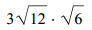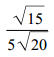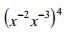### Day 08 - Students as Teachers - 08.27.14

 Bell RingerReviewExponent PropertiesProduct of PowersPower of a PowerPower of a ProductQuotient of PowersPower of a QuotientZero ExponentNegative ExponentsRadical PropertiesProduct of Square RootsQuotient of Square RootsRationalizing the DenominatorRational ExponentsExponential Growth and DecayLessonStudents as the Teachers!Exit TicketSubmit Exit Ticket problems from the board. Lesson Objective(s) How are exponent properties applied to simplify problems?How are radical properties used to simplify radical expressions and solve radical equations? Standard(s) N.RN.1  Explain how the definition of the meaning of rational exponents follows from extending the properties of integer exponents to those values, allowing for a notation for radicals in terms of rational exponents. N.RN.2  Rewrite expressions involving radicals and rational exponents using the properties of exponents. A.CED.1 Create equations and inequalities in one variable and use them to solve problems.Include equations arising from linear and quadratic functions, and exponential functions. Mathematical Practice(s) #2: Reason abstractly and quantitatively Students will use concrete examples of numerical manipulation to examine closure of rational and irrational numbers. For example, students will use numeric examples of sums and products of rational numbers to generalize the closure of rational numbers under addition and multiplication. #4: Model with mathematics Students will create equations using rational or radical expressions to represent mathematical models of real-world situations like interest rates or depreciation.